Courses

# Percentile Booster - Quant: Time & Work CAT Notes | EduRev

## CAT : Percentile Booster - Quant: Time & Work CAT Notes | EduRev

The document Percentile Booster - Quant: Time & Work CAT Notes | EduRev is a part of CAT category.
All you need of CAT at this link: CAT

1. Darbar Toy Company has to go through the following stages for the launch of a new toy: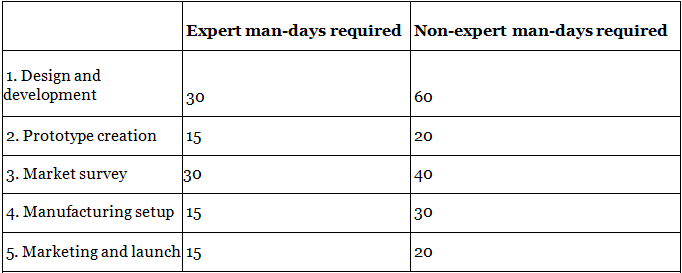The profile of the company's manpower is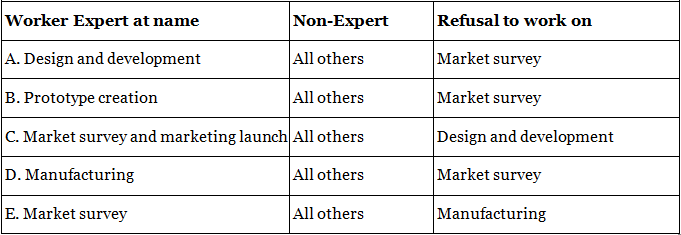Given this situation, the minimum number of days in which the company can launch a new toy going through all the stages is
(a) 40 days
(b) 40.5 days
(c) 45 days
(d) 44 days
Correct Option: B
Solution:
Interpretation of the first row of the first table in the question: Design and Development requires 30 expert mandays or 60 non-expert man-days. Hence, work done in 1 expert man-day = 3.33% and work done in 1 non-expert man-day = 1.66%. Further, from the second table, it can be interpreted that: A is an expert at design and development. Hence, his work rate is 3.33% per day and B, D and E are ready to work as non-experts on design and development, hence their work rate is 1.66% per day each. Thus, in 1 day the total work will be A + B + D + E = 3.33 + 1.66 + 1.66 + 1.66 = 8.33% work. Thus, 12 days will be required to finish the design and development phase.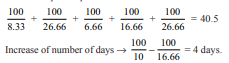[This happens since the work rate will drop from 16.66% to 10% due to A and C's refusal to work.] Find out the work done by each of the 5 workers.

2. Darbar Toy Company has to go through the following stages for the launch of a new toy:The profile of the company's manpower isIf A and C refuse to have anything to do with the manufacturing set up. the number of days by which the project will get delayed will be
(a) 5 days
(b) 4 days
(c) 3 days
(d) 6 days
Correct Option: B
Solution:
Interpretation of the first row of the first table in the question: Design and Development requires 30 expert mandays or 60 non-expert man-days. Hence, work done in 1 expert man-day = 3.33% and work done in 1 non-expert man-day = 1.66%. Further, from the second table, it can be interpreted that: A is an expert at design and development. Hence, his work rate is 3.33% per day and B, D and E are ready to work as non-experts on design and development, hence their work rate is 1.66% per day each. Thus, in 1 day the total work will be A + B + D + E = 3.33 + 1.66 + 1.66 + 1.66 = 8.33% work. Thus, 12 days will be required to finish the design and development phase.[This happens since the work rate will drop from 16.66% to 10% due to A and C's refusal to work.] Find out the work done by each of the 5 workers.

3. A pipe can fill a tank in x hours and another can empty it in y hours. If the tank is 1/3rd full then the number of hours in which they will together fill it in is
(a) (3xy)/2(y - x)
(b) (3xy)/(y - x)
(c) (xy)/3(y - x)
(d) (2xy)/3(y - x)
Correct option: D
Solution:
To solve this question first assume the values of x and y (such that x < y). If you take x as 10 hours and y as 15 hours, you will get a net work of 3.33% per hour. At this rate it will take 20 hours to fill the tank from one third full. Using this condition try to put these values of x and y into the options to check the values. For instance option (a) gives the value as 3 x 10 x 15/10 = 45 which is not equal to 20

4. Dev and Tukku can do a piece of work in 45 and 40 days respectively. They began the work together, but Dev leaves after some days and Tukku finished the remaining work in 23 days. After how many days did Dev leave
(a) 7 days
(b) 8 days
(c) 9 days
(d) 11 days
Correct option: C
Solution:
n(1/45 + 1/40) + 23/40 = 1 → n = 9.

5. A finishes 6/7th of the work in 2z hours, B works twice as fast and finishes the remaining work. For how long did B work?
(a) (2/3)z
(b) (6/7)z
(c) (6/49) z
(d) (3/18) z
Correct option: D
Solution:
Since A finishes 6/7th of the work in 2z hours . B would finish 12/7 of the work in 2z hours. Thus, to do 1/7th of the work (which represents the remaining work), B would require 2z/12 = z/6 hours. Option (d) is correct.

6. A and B completed a work together in 5 days. Had A worked at twice the speed and B at half the speed, it would have taken them four days to complete the job. How much time would it take for A alone to do the work?
(a) 10 days
(b) 20 days
(c) 25 days
(d) 15 days
Correct Option: A
Solution:
A + B = 20% of the work. Use trial and error with the options to get the answer. Checking for option (a), A = 10% and B = 10%. If A doubles his work and B halves his work rate, the total work in a day would become A = 20, B = 5. This would mean that the total work would get completed in 4 days which is the required condition that needs to be matched if the option is to be correct. Hence, this option is correct.

7. Three cooks have to make 80 idlis. They are known to make 20 pieces every minute working together. The first cook began working alone and made 20 pieces having worked for sometime more than three minutes. The remaining part of the work was done by the second and the third cook working together. It took a total of 8 minutes to complete the 80 idlis. How many minutes would it take the first cook alone to cook 160 idlis for a marriage party the next day?
(a) 16 minutes
(b) 24 minutes
(c) 32 minutes
(d) 40 minutes
Correct Option: C
Solution:
From the condition of the problem and a little bit of trial and error we can see that the first cook worked for 4minutes and the 2nd and 3rd cooks also worked for 4minutes. As 4(A) + 4(B + C) = 4(A + B + C) and we know that A + B + C = 20 idlis per minute. Thus, the first cook make 20 idlis in 4 minutes. To make 160 idlis he would take 32 minutes.

8. Each of A, B and C, working alone, can complete a piece of work in 10 days, 20 days and 30 days, respectively. They work in such a manner that on 1st day A and B work, on 2nd day B and C work and on 3rd day C and A work. If work is continued in the same sequence, then on which day the work will be completed and who will complete it?
(a) 8th, B and C
(b) 9th, B and C
(c) 9th, C and A
(d) 7th, A and B
Correct Option: C
Solution:
On 1st day part of work complete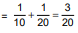On 3rd day part of work completed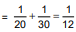In three days part of work completed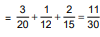In 6 days part of work completed = 22/30
Remaining work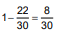On 7th day, part of work remained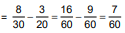On 8th day, part of work remained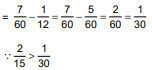∴ The work will be completed on 9th day by C and A.

9. A tank of capacity 25 litres has an inlet and an outlet tap. If both are opened simultaneously, the tank is filled in 5 minutes. But if the outlet flow rate is doubled and taps opened the tank never gets filled up. Which of the following can be outlet flow rate in litres/min?
(a) 2
(b) 6
(c) 4
(d) 3
Correct option: B
Solution:
The net inflow when both pipes are opened is 5 litres a minute. The outlet flow should be such that if its rate is doubled the net inflow rate should be negative or 0. Only an option greater than or equal to ‘5’ would satisfy this condition. Option (b) is the only possible value

10. Each of Atul, Yogesh and Riyaz can complete a piece of work in 12 days, 36 days and 54 days respectively. They started the work together, but Atul left after working for 6 days and Yogesh left 8 days before the completion of the work. In how many days was the work completed?
(a) 16
(b) 17
(c) 20
(d) 15 3/5
Correct Option: D
Solution:
Let us assume that the work can be completed in n days. Thus,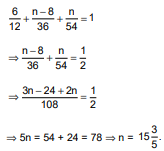11. Two cigarette smokers A and B take puffs on the same cigarette alternately starting with A. A takes a puff for 2 s and then B takes a puff for 3 s. Rate of burning while taking a puff is 3 mm/s and while not puffing is 1 mm/s. After how much time will the cigarette be completely burnt, if its length is 84 mm and the gap between any two consecutive puffs is 3s?
(a) 40 sec
(b) 35 sec
(c) 44 sec
(d) 36 sec
Correct Option: C
Solution:
As A starts puffing, the length of the cigarette th gets burnt in one cycle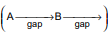= (2 x 3) + 3 + (3 x 3) + 3 = 6 + 3 + 9 + 3 = 21 mm. 84 mm will get burnt in four such cycles.
(∵ 84/21 = 4)

Time taken in one cycle = 2 + 3 + 3 + 3 = 11s. So. total time = 4 x 11 = 44s.

12. Read the following and answer the questions that follow. A set of 10 pipes (set X) can fill 70% of a tank in 7 minutes. Another set of 5 pipes (set Y) fills 3/8 of the tank in 3 minutes. A third set of 8 pipes (set Z) can empty 5/10 of the tank in 10 minutes.
How many minutes will it take to fill the tank if all the 23 pipes are opened at the same time?
(a) 5 minutes
(b)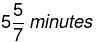(c) 6 minutes
(d)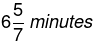Correct Option: B
Solution:
If all the 23 pipes are opened the per minute rate will be: 10 + 12.5 – 5 = 17.5%  Option (b) is correct.

13. Read the following and answer the questions that follow. A set of 10 pipes (set X) can fill 70% of a tank in 7 minutes. Another set of 5 pipes (set Y) fills 3/8 of the tank in 3 minutes. A third set of 8 pipes (set Z) can empty 5/10 of the tank in 10 minutes.
If only half the pipes of set X are closed and only half the pipes of set Y are open and all other pipes are open, how long will it take to fill 49% of the tank?
(a) 16 minutes
(b) 13 minutes
(c) 7 minutes
(d) None of these
Correct Option: D
Solution:
Set X will do 5 % per minute and Set Y will do 6.25% per minute, while set Z will do 5% per minute (negative work). Hence, Net work will be 6.25% per minute. To fill 49% it will take slightly less than eight minutes and the value will be a fraction. None of the first three options matches this requirement. Hence, the answer will be (d).

14. Read the following and answer the questions that follow. A set of 10 pipes (set X) can fill 70% of a tank in 7 minutes. Another set of 5 pipes (set Y) fills 3/8 of the tank in 3 minutes. A third set of 8 pipes (set Z) can empty 5/10 of the tank in 10 minutes.
If 4 pipes are closed in set Z, and all others remain open, how long will it take to fill the tank?
(a) 5 minutes
(b) 6 minutes
(c) 7 minutes
(d) 7.5 minutes
Correct Option: A
Solution:
If 4 of the taps of set Z are closed, the net work done by Set Z would be –2.5% while the work done by Sets X and Y would remain 10% and 12.5% respectively. Thus, the total work per minute would be 20% and hence the tank would take 5 minutes to fill up

15. Read the following and answer the questions that follow. A set of 10 pipes (set X) can fill 70% of a tank in 7 minutes. Another set of 5 pipes (set Y) fills 3/8 of the tank in 3 minutes. A third set of 8 pipes (set Z) can empty 5/10 of the tank in 10 minutes.
If the tank is half full and set X and set Y are closed, how many minutes will it take for set Z to empty the tank if alternate taps of set Z are closed.
(a) 12 minutes
(b) 20 minutes
(c) 40 minutes
(d) 16 minutes
Correct Option: B
Solution:
Again if we close 4 taps of set Z, the rate of emptying by set Z would be 2.5% per minute. A half filled tank would contain 50% of the capacity and hence would take 50/2.5 = 20 minutes to empty

16. Read the following and answer the questions that follow. A set of 10 pipes (set X) can fill 70% of a tank in 7 minutes. Another set of 5 pipes (set Y) fills 3/8 of the tank in 3 minutes. A third set of 8 pipes (set Z) can empty 5/10 of the tank in 10 minutes.
If one pipe is added for set X and set Y and set Z’s capacity is increased by 20% on its original value and all the taps are opened at 2.58 p.m., then at what time does the tank get filled? (If it is initially empty.)
(a) 3.05 p.m.
(b) 3.04 p.m.
(c) 3.10 p.m.
(d) 3.03 p.m.
Correct Option: D
Solution:
The rate per minute with the given changes (in percentage terms) would be: Set X =11%, Set Y = 15% and Set Z = –6%. Hence, the net rate = 11 + 15 – 6 = 20% per minute and it would take 5 minutes for the tank to fill. If all pipes are opened at 7:58, the tank would get filled at 3:03

17. Two forest officials in their respective divisions were involved in the harvesting of tendu leaves. One division had an average output of 21 tons from a hectare and the other division, which had 12 hectares of land less, dedicated to tendu leaves, got 25 tons of tendu from a hectare. As a result, the second division harvested 300 tons of tendu leaves more than the first. How many tons of tendu leaves did the first division harvest?
(a) 3150
(b) 3450
(c) 3500
(d) 3600
Correct Option: A
Solution:
25 (n – 12) = 21 n + 300. Solving this equation, n = 150. Hence, the first division harvest 3150 tons.

18. It takes six days for three women and two men working together to complete a work. Three men would do the same work five days sooner than nine women. How many times does the output of a man exceed that of a woman?
(a) 3 times
(b) 4 times
(c) 5 times
(d) 6 times
Correct Option: C
Solution:
Solve this using options. If we check for option (c), i.e., the work of a man exceeds the work of a woman by 5 times, we would get the following thought process: Total work = 6 days * (3 women + 2 men) = 18 woman days + 12 man days = 18 woman days + 60 woman days = 78 woman days. Thus, 9 women would take 78/9 days = 8.66 days and hence 3 men should do the same work in 3.66 days. This translates to 3* 3.66 = 10 man days or 50 woman days which is incorrect as the number of woman days should have been 78. Thus, we can reject this option. If we check for option (d), i.e., the work of a man exceeds the work of a woman by 6 times, we would get the following thought process: Total work = 6 days * (3 women + 2 men) = 18 woman days + 12 man days = 18 woman days + 72 woman days = 90 woman days. Thus, 9 women would take 90/9 days = 10 days and hence 3 men should do the same work in 5 days. This translates to 3 * 5 = 15 man days or 90 woman days which is correct as the number of woman days should be 90.

19. Two inlet pipes can seperately fill a tank in 20 minutes and 15 minutes respectively and a waste pipe can empty the completely filled tank in 12 minutes. On a certain day, the two inlet pipes are turned on simultaneously to fill the empty tank. But after 9 minutes it was found that the waste pipe was also left opened, it was closed immediately. How much more time is required to fill the tank completely?
(a) 5.5 min
(b) 5 min
(c) 6 min

(d) 6.2 min
Correct Option: D
Solution: Let total work be 60 units. 1st tap fills 3units per minute, 2nd tap fills 4 units per minute and waste pipe empties 5 units per minute. So, when opened together all three will fill (3 + 4 – 5) = 2 units per min. Hence in first 9 min, 18 units of tank is filled. Since the waste pipe is closed ∴ The remaining 42 units will require 42 /3 +4  = 6 min to fill.

20. In a factory's total daily wages of 20 men, 30 women and 36 children is Rs. 78. The ratio of work done by a man, a woman and a child in a day is 3 : 2 : 1 respectively. What will be the total wages of 15 men, 21 women and 30 children for 18 weeks?
(a) Rs. 7,371
(b) Rs. 9,585
(c) Rs. 6,956
(d) Rs. 5,000
Correct Option: A
Solution:
Let the work done by a man, a woman and a child in a day be m, w and c respectively. Then, m = 3c and w = 2c ∴ 20m + 30w + 36c = 60c + 60c + 36c = 156c Total units of work done in second case = (15m + 21w + 30c) × 7 × 18 = (45c + 42c + 30c) × 7 × 18 = 117c × 7 × 18 Hence, the required total ways 78 /156c = × 117c × 7 × 18 = Rs. 7,371.

Offer running on EduRev: Apply code STAYHOME200 to get INR 200 off on our premium plan EduRev Infinity!

,

,

,

,

,

,

,

,

,

,

,

,

,

,

,

,

,

,

,

,

,

;